# NEET Important Chapter - Electromagnetic Induction

Get plus subscription and access unlimited live and recorded courses## Electromagnetic Induction - An Important Concept for NEET

From previous chapters, we know that current produces magnetic fields. Is the reverse of this true? Can a magnetic field produce electric current? The answer is yes. For a very long time, electricity and magnetism were considered a separate field but it is now known that they are interrelated.

It is found that currents are produced in closed coils when placed in a varying magnetic field. The phenomenon in which current is induced by a changing magnetic field is known as Electromagnetic Induction. The current thus produced is called the induced current. If the current is produced, it must be due to some emf produced in the circuit, which is known as induced emf.

In this chapter, students will learn about the central principle of electromagnetic induction i.e. Faraday’s laws. Students will also learn about Lenz's law, which helps to predict the direction of induced current.

Going further in this chapter, students will also learn about the construction and working of devices based on electromagnetic induction such as power generators, dynamos, transformers, etc.

Now, let us move on to the important concepts and formulae related to the NEET exam along with a few solved examples.

Important Topics of Electromagnetic Induction

• Electromagnetic Induction

• Magnetic Flux

• Lenz’s Law

• Motional Electromotive Force

• Self-inductance

• Mutual Inductance

• Growth and Decay of Current in an L-R Circuit

• Oscillations in L-C Circuit

### Solved Examples

1. The current in a long current carrying wire is I = 2t. A conducting loop is placed to the right of this wire. Find magnetic flux $\phi_B$ passing through the loop.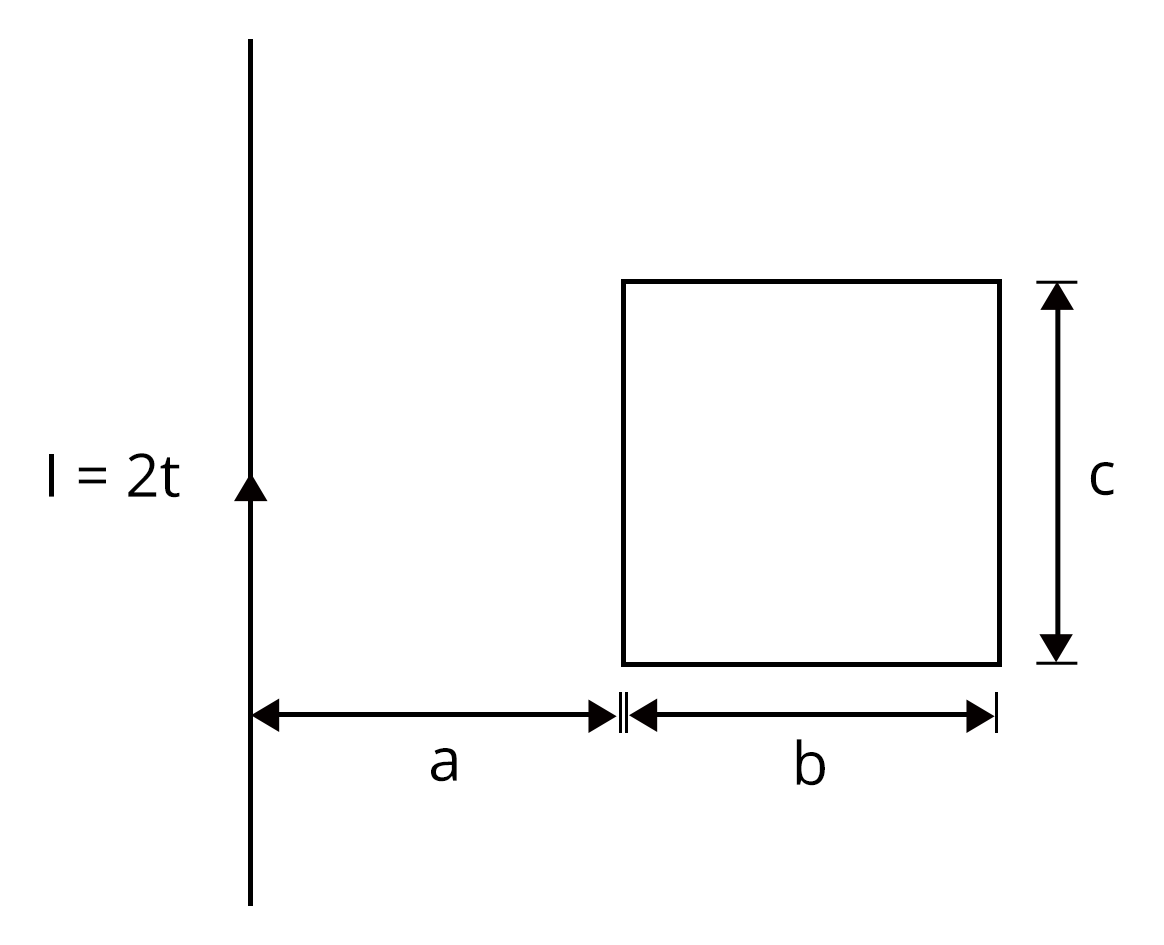Sol:

In this situation, there is no motion of the conductor and the magnetic field changes with the distance from the current carrying conductor. So, the magnetic flux will be obtained by using an integration method.

Consider an element of thickness, dx at a distance x from the wire as shown in the figure.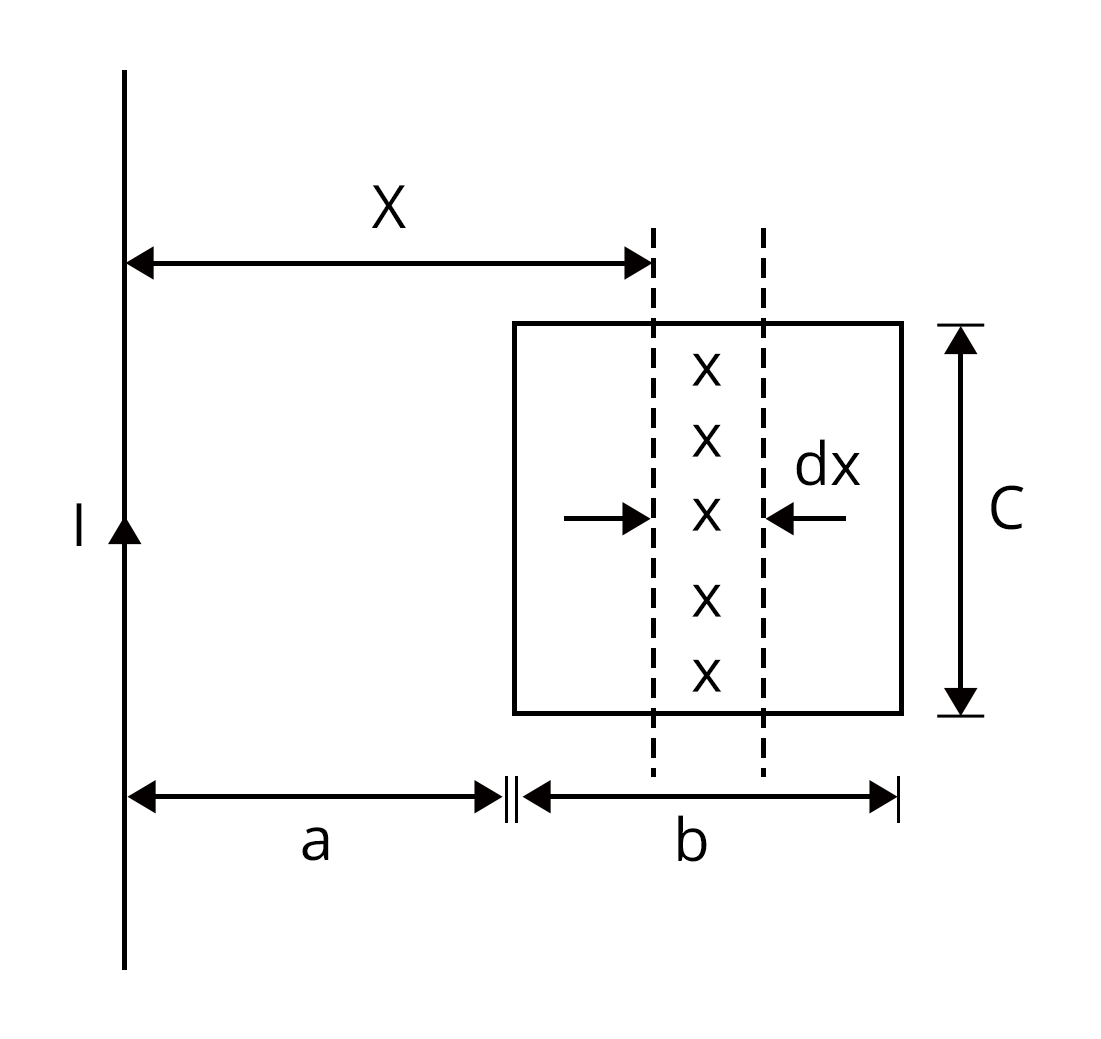At distance, x magnetic field (B) is

$B = \dfrac{\mu_0}{2\pi}\dfrac{I}{x}$

Now, let us find the area of the strip

$dS = c(dx)$

Therefore, a small magnetic flux passing through the loop will be

$d\phi_B= BdS\cos0°$

$d\phi_B= \dfrac{\mu_0}{2\pi}\dfrac{I}{x}cdx$

The total magnetic flux is

$\phi_B= \int_{a}^{a+b} d\phi_B$

$\phi_B= \int_{a}^{a+b} \dfrac{\mu_0Ic}{2\pi}\dfrac{dx}{x}$

$\phi_B=\dfrac{\mu_0Ic}{2\pi}\ln\lgroup\dfrac{a+b}{a}\rgroup$

Also, it is given that I = 2t

$\phi_B=\dfrac{\mu_0Itc}{\pi}\ln\lgroup\dfrac{a+b}{a}\rgroup$

Hence, the magnetic flux passing through the loop is $\dfrac{\mu_0Itc}{\pi}\ln\lgroup\dfrac{a+b}{a}\rgroup$

Key point: In such cases where there is no motion and magnetic field is varying as a function of distance, the integration method comes in handy.

2. A square loop of side l having resistance R moves with constant velocity v, through a constant magnetic field as shown in figure. Find the magnitude and direction of induced current in the situation when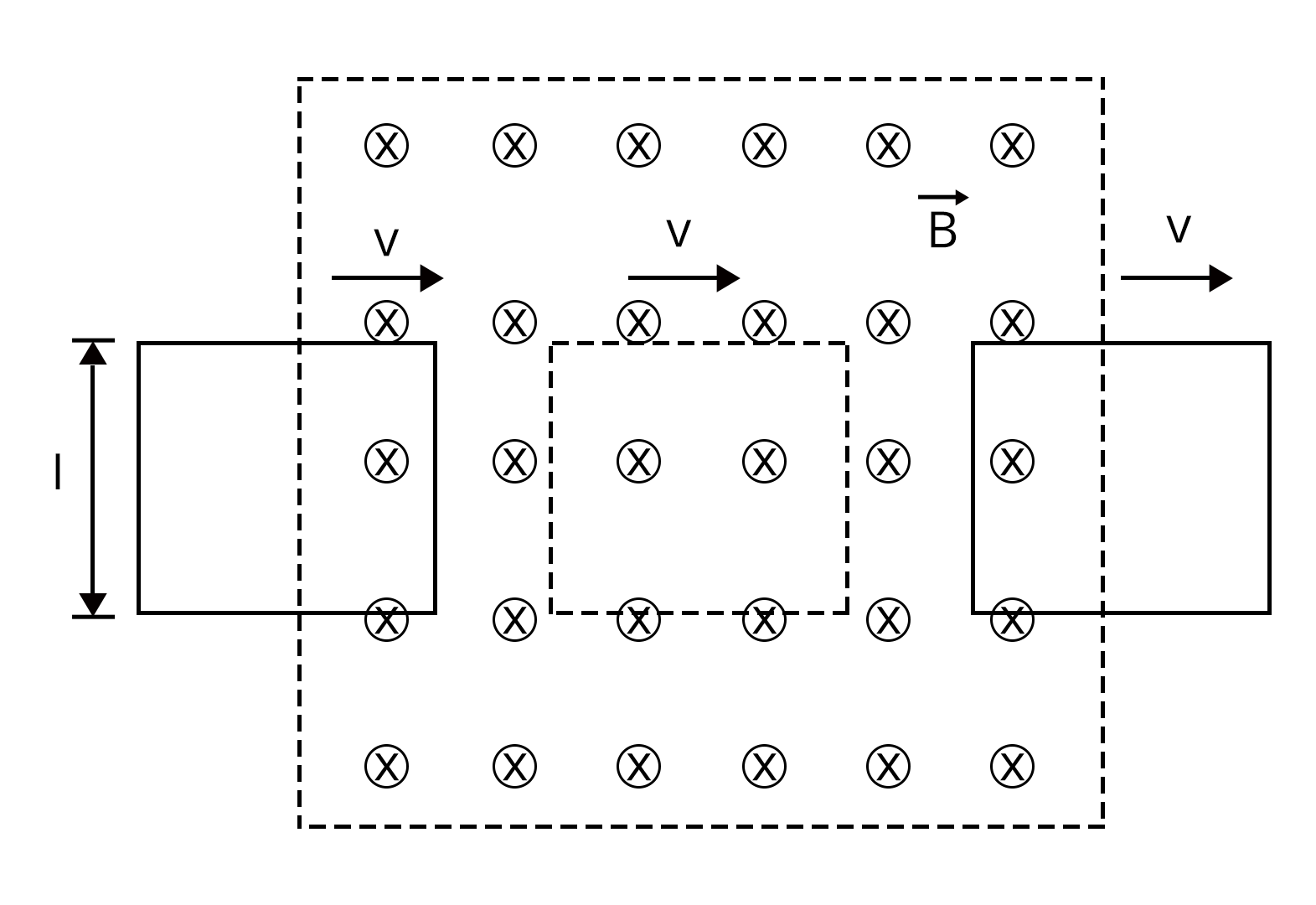1. The loop enters into the magnetic field.

2. It is moving in a magnetic field.

3. It is coming out of the magnetic field.

Sol:

1. Here the side ad is outside the field. Therefore, no magnetic flux will be induced in side ad. Now, sides ab and cd are parallel to the velocity of the loop and no current is induced when the velocity is parallel to the velocity of the loop. So, the emf will only be induced in the side cb as shown in figure.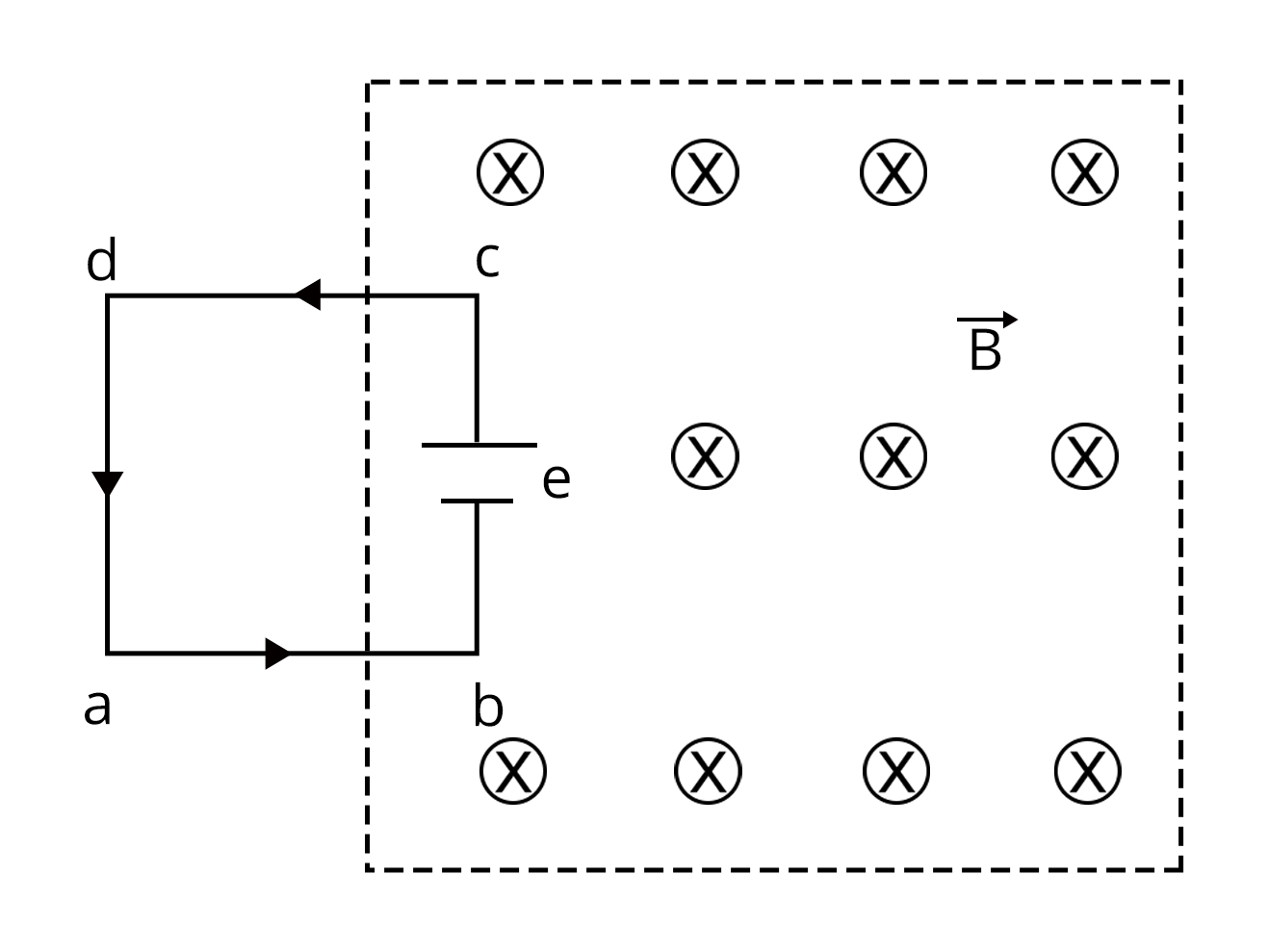Hence, net emf induced will be $\varepsilon= Blv$

The current in the loop $I = \dfrac{\varepsilon}{R}$ = $\dfrac{Blv}{R}$. The current will be counterclockwise

2. When the loop is completely submerged in the field, there is no change in the flux w.r.t. time. So, there will not be an induction of current.

3. When the loop starts coming out of the magnetic field. The emf will be induced in side ad only. Using Lenz’s law, the direction of current will be clockwise.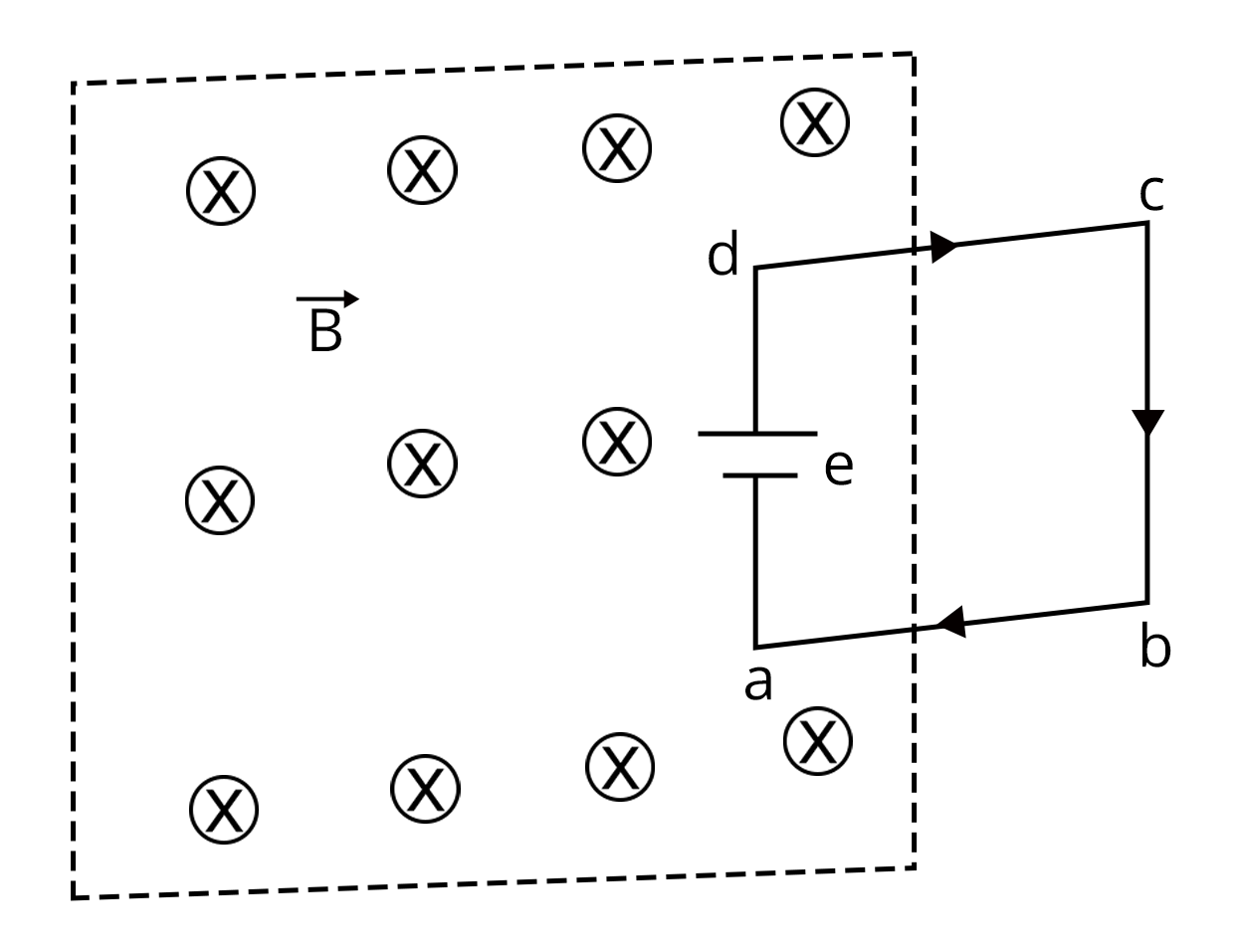Hence, the net emf induced will be $\varepsilon= Blv$

The current in the loop $I = \dfrac{\varepsilon}{R}$ = $\dfrac{Blv}{R}$.

Key Point: Always use Lenz’s law to find the direction of induced current.

### Previous Year Questions from NEET Paper

1. The magnetic flux linked with a coil (in Wb) is given by the equation $\phi= 5t^2+3t+16$. The magnitude of induced emf in the coil at the fourth second will be  (NEET 2020)

1. 33 V

2. 43 V

3. 108 V

4. 10 V

Sol:

The magnetic flux linked with the coil is given as

$\phi= 5t^2+3t+16$

The magnitude of the induced emf is

$e= \dfrac{\text{d}\phi}{\text{d}t} = e = \dfrac{d}{dt}5t^2+3t+16$

At t = 3s, $e_3$ = 33V,

At t = 4s, $e_4$ = 43V,

Therefore, the induced emf will be:

$e= e_4-e_3$ = 10 V

Hence, the correct answer is option (d)

Trick: The rate of change of magnetic flux is emf induced in the conductor.

2. A wheel with 20 metallic spokes, each 1 m long, is rotated with the speed of 120 rpm in a plane perpendicular to a magnetic field of 0.4 G. The induced emf between axle and rim of the wheel will be (1G = $10^{-4}$T) (NEET 2020)

1. $2.51 \times10^{-4} V$

2. $2.51 \times10^{-5} V$

3. $4.0 \times10^{-5} V$

4. $2.51 V$

Sol:

Given,

Magnetic field (B) =  0.4 G = $0.4 \times10^{-4} T$,

Frequency (f) = 120 rpm = $\dfrac{120}{60}$ rps = 2 Hz,

The induced emf between the axle and the rim of the wheel is given as

$|e|= \dfrac{1}{2}Bl^{2}\omega$

$|e|= \dfrac{1}{2}Bl^{2}(2\pi f)$ = $2.51 \times10^{-4} V$

Option (a) is the correct answer.

Trick: Rotational emf is given by $|e|= \dfrac{1}{2}Bl^{2}\omega$

### Practice Questions

1. A coil formed by wrapping 50 turns of a wire in the shape of a square is positioned in a magnetic field so that the normal to the plane of the coil makes an angle of 30°, with the direction of the field. When the magnetic field is increased uniformly from 200 µT to 600 µT in 0.4 s, an emf of magnitude 80.0 mV is induced in the coil. What is the total length of the wire? (Ans. 272 m)

2. A square frame with side a and a long straight wire carrying a current i are located in the same plane as shown in the figure. The frame translates to the right with a constant velocity v. Find the emf induced in the frame as a function of distance x. (Ans. $e = \dfrac{\mu_0}{4\pi}\dfrac{2ia^2v}{x(x+a)}$ )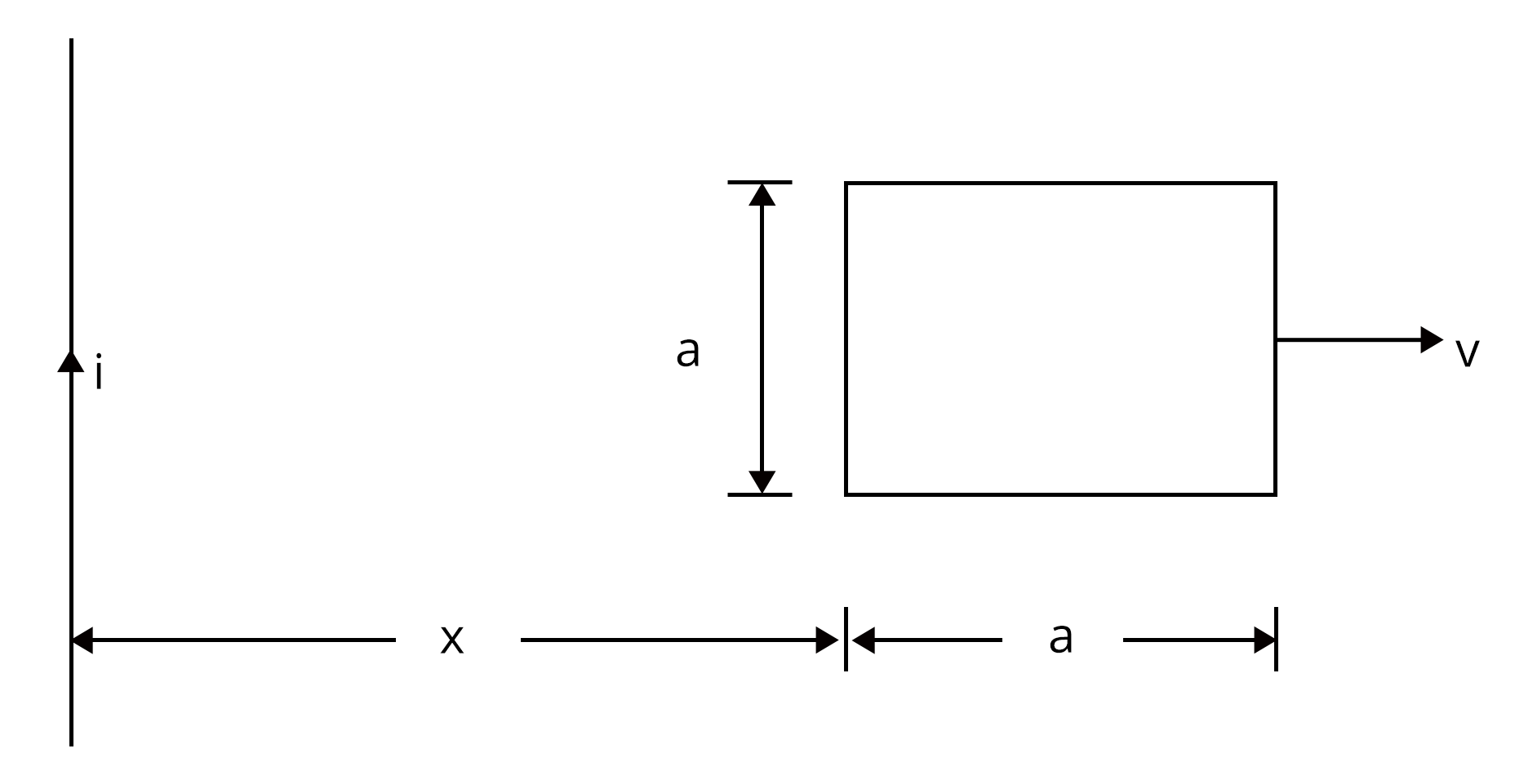### Conclusion

In this article, we have provided important information regarding the chapter Electromagnetic Induction such as important concepts, formulae, etc. Students should work on more solved examples for securing good grades in the NEET 2022 exam.

See More## NEET Important Dates

View All Dates
NEET 2022 exam date and revised schedule have been announced by the NTA. NEET 2022 will now be conducted on 17-July-2022, and the exam registration closes on 20-May-2022. You can check the complete schedule on our site. Furthermore, you can check NEET 2022 dates for application, admit card, exam, answer key, result, counselling, etc along with other relevant information.
See More
View All Dates## NEET Information

Application Form
Eligibility Criteria
Reservation Policy
NTA has announced the NEET 2022 application form release date on the official website https://neet.nta.nic.in/. NEET 2022 Application Form is available on the official website for online registration. Besides NEET 2022 application form release date, learn about the application process, steps to fill the form, how to submit, exam date sheet etc online. Check our website for more details.## NEET 2022 Study Material

View NEET Syllabus in Detail
View NEET Syllabus in Detail## NEET 2022 Study Material

View all study material for NEET
All
Physics
Chemistry
Biology
See All## NEET Question Papers## NEET 2022 Book Solutions and PDF Download

View all NEET Important Books
Biology
NCERT Book for Class 12 Biology
Physics
NCERT Book for Class 12 Physics
Chemistry
NCERT Book for Class 12 Chemistry
Physics
H. C. Verma Solutions
See All## NEET Mock Tests

View all mock tests
"NEET 2022 free online mock test series for exam preparation are available on the Vedantu website for free download. Practising these mock test papers of Physics, Chemistry and Biology prepared by expert teachers at Vedantu will help you to boost your confidence to face the NEET 2022 examination without any worries. The NEET test series for Physics, Chemistry and Biology that is based on the latest syllabus of NEET and also the Previous Year Question Papers."
See More## NEET 2022 Cut Off

NEET Cut Off
NTA is responsible for the release of the NEET 2022 cut off score. The qualifying percentile score might remain the same for different categories. According to the latest trends, the expected cut off mark for NEET 2022 is 50% for general category candidates, 45% for physically challenged candidates, and 40% for candidates from reserved categories. For the general category, NEET qualifying marks for 2021 ranged from 720-138 general category, while for OBC/SC/ST categories, they ranged from 137-108 for OBC, 137-108 for SC and 137-108 for ST category.
See More## NEET 2022 Results

The NEET 2022 result is published by NTA on https://neet.nta.nic.in/ in the form of a scorecard on 7-Sep-2022. The scorecard will include the roll number, application number, candidate's personal details, and the percentile, marks, and rank of the candidate. Only those candidates who achieve the NEET cut-off will be considered qualified for the exam.
See More
Rank List
Counselling
Cutoff
NEET 2022 state rank lists will be released by the state counselling committees for admissions to the 85% state quota and to all seats in private medical and dental colleges. NEET 2022 state rank lists are based on the marks obtained in entrance exams. Candidates can check the NEET 2022 state rank list on the official website or on our site.## NEET Top Colleges

View all NEET 2022 Top Colleges
Want to know which Engineering colleges in India accept the NEET 2022 scores for admission to Engineering? Find the list of Engineering colleges accepting NEET scores in India, compiled by Vedantu. There are 1622 Colleges that are accepting NEET. Also find more details on Fees, Ranking, Admission, and Placement.
See More## FAQs on NEET Important Chapter - Electromagnetic Induction

FAQ

1. What is the weightage of the Electromagnetic Induction in NEET?

Nearly 2-3 questions arise in the exam from this chapter almost every year. Hence, students should definitely lay more emphasis on the chapter of Electromagnetic Induction.

2. What are the key points that need to be practised for solving questions from Electromagnetic Induction?

Students should concentrate on understanding Faraday’s and Lenz’s law thoroughly while practising for solving questions from Electromagnetic Induction.

3. Are previous year questions enough for NEET?

To score 640+ in NEET, NCERT (both 11th and 12th) and previous year NEET papers are sufficient. Solving previous 10 years NEET examination papers offers a tremendous advantage because 6-7 questions with identical alternatives are guaranteed to be repeated every year.## Notice board

News
BlogTrending pages